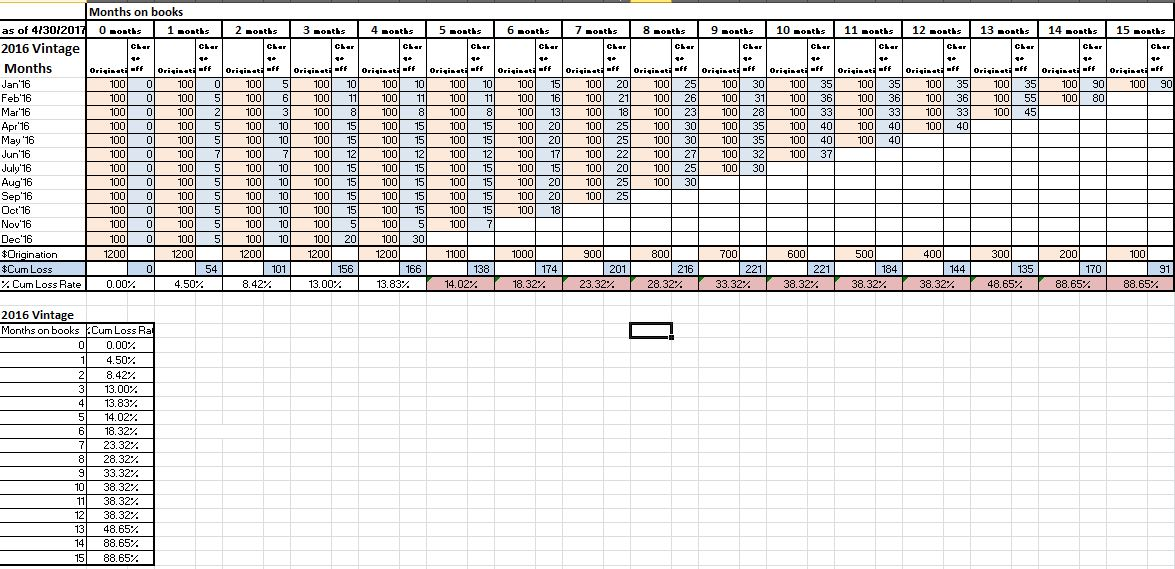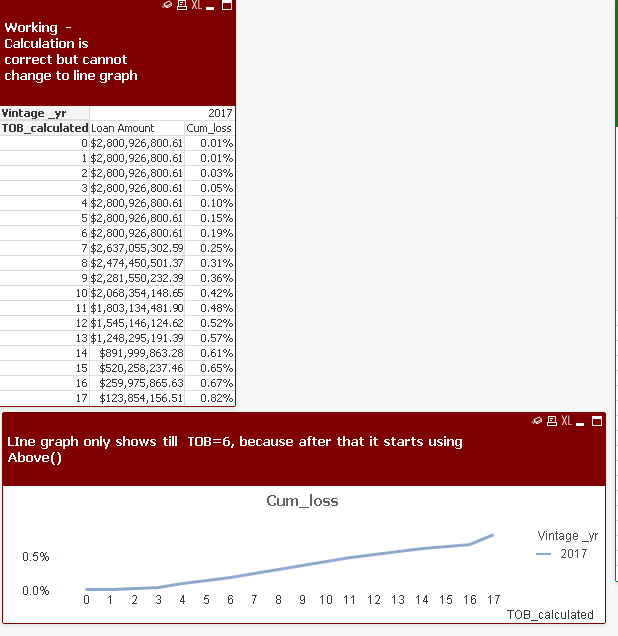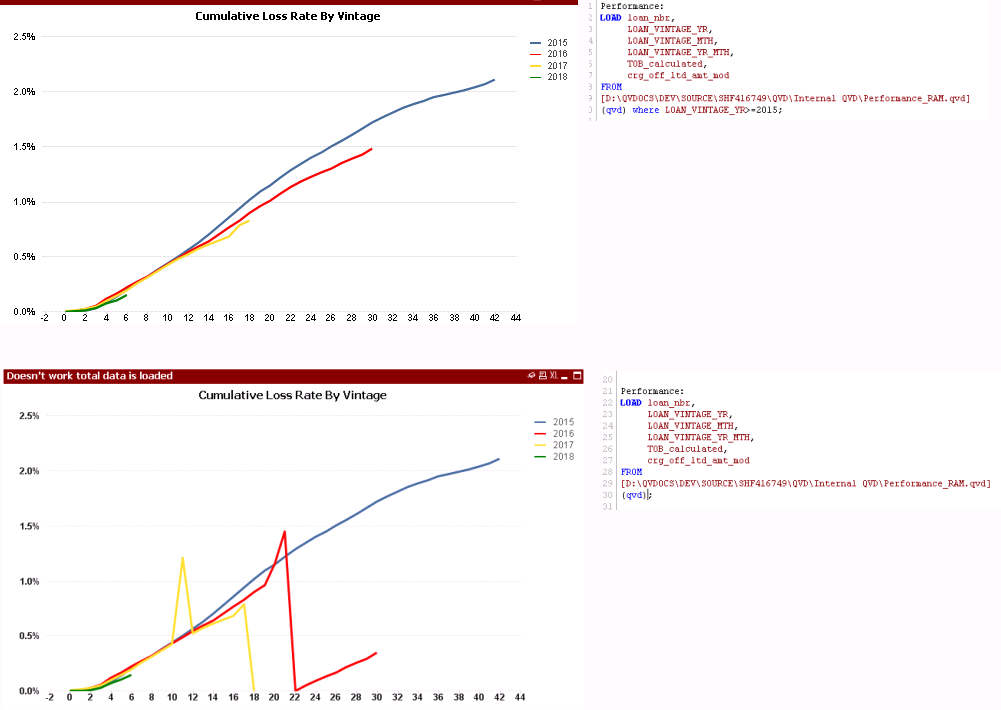# New to QlikView

Discussion board where members can get started with QlikView.

Announcements
Attend QlikWorld 2020 and hear keynote speaker, Malcolm Gladwell. Register by February 29th to save \$200. Learn More
Contributor

## Qlikview pivot table - Cumulative calculation without using Above() and Rangesum() functionsI have the attached summary loan data consisting of originated month, total originated \$ in the month and total cumulative Charge off \$ from month to month. expected output is the second table where cumulative loss rate for the entire loan Vintage is shown. I have developed this pivot using above() and rangesum() functions but when I convert the pivot table to a line chart, the above() and rangesum() wont retain their functionality and I see completely different %'s. It would be soo easy to convert these pivot into graphs instead of manually exporting the pivot to excel and graphing

Tags (4)
1 Solution

Accepted Solutions
HighlightedMVP

## Re: Qlikview pivot table - Cumulative calculation without using Above() and Rangesum() functions

Try this

Aggr(if(TOB_calculated <= MAX_TOB_VINTAGE_YR,

if(MAX_LOAN_VINTAGE_MTH>=12 or TOB_calculated=0,

(sum(crg_off_ltd_amt_mod)/SUM (ORG_PRIN_AMT)),

if(rank(-if(MAX_LOAN_VINTAGE_MTH<12,TOB_calculated))=1,

( above(sum(crg_off_ltd_amt_mod)/SUM (ORG_PRIN_AMT))

+

(sum(crg_off_ltd_amt_mod)-above(if(MAX_LOAN_VINTAGE_MTH>12, sum(crg_off_ltd_amt_mod), sum(if(MAX_TOB_VINTAGE_YR_MTH > TOB_calculated, crg_off_ltd_amt_mod)))))/SUM (ORG_PRIN_AMT)),

RangeSum(Above(Column(1)),(sum(crg_off_ltd_amt_mod)-above(if(MAX_LOAN_VINTAGE_MTH>12, sum(crg_off_ltd_amt_mod), sum(if(MAX_TOB_VINTAGE_YR_MTH > TOB_calculated, crg_off_ltd_amt_mod)))))/SUM (ORG_PRIN_AMT)))

),

null()), LOAN_VINTAGE_YR, TOB_calculated)15 Replies
Highlighted
Honored Contributor

## Re: Qlikview pivot table - Cumulative calculation without using Above() and Rangesum() functions

May be try your expression like this:

Dimension

Month

Expression as Below

RangeSum(Above(COUNT(Product),0,RowNo()))/RangeSum(Above(COUNT({<Product = , Month = {'>=\$(=Min(Month))<=\$(=Max(Month))'}>}Product),0,RowNo()))

Or

aggr(rangesum(above(Count([ID ordine]),0,RowNo()))/sum(total <[\$Production Year]> Qty),([\$Production Year],(NUMERIC, ASCENDING)),([Claim Year],(NUMERIC, ASCENDING)))

HighlightedMVP

## Re: Qlikview pivot table - Cumulative calculation without using Above() and Rangesum() functions

Would you be able to share the app where you have already done this in a table? It might be easy to see what you have and then help you convert this into a line chart in the same app

Best,

Sunny

Highlighted
Contributor

## Re: Qlikview pivot table - Cumulative calculation without using Above() and Rangesum() functions

Attached QVW to the original post. thanks

HighlightedMVP

## Re: Qlikview pivot table - Cumulative calculation without using Above() and Rangesum() functions

Try this

Aggr(if(TOB_calculated <= MAX_TOB_VINTAGE_YR,

if(MAX_LOAN_VINTAGE_MTH>=12 or TOB_calculated=0,

(sum(crg_off_ltd_amt_mod)/SUM (ORG_PRIN_AMT)),

if(rank(-if(MAX_LOAN_VINTAGE_MTH<12,TOB_calculated))=1,

( above(sum(crg_off_ltd_amt_mod)/SUM (ORG_PRIN_AMT))

+

(sum(crg_off_ltd_amt_mod)-above(if(MAX_LOAN_VINTAGE_MTH>12, sum(crg_off_ltd_amt_mod), sum(if(MAX_TOB_VINTAGE_YR_MTH > TOB_calculated, crg_off_ltd_amt_mod)))))/SUM (ORG_PRIN_AMT)),

RangeSum(Above(Column(1)),(sum(crg_off_ltd_amt_mod)-above(if(MAX_LOAN_VINTAGE_MTH>12, sum(crg_off_ltd_amt_mod), sum(if(MAX_TOB_VINTAGE_YR_MTH > TOB_calculated, crg_off_ltd_amt_mod)))))/SUM (ORG_PRIN_AMT)))

),

null()), LOAN_VINTAGE_YR, TOB_calculated)Highlighted
Contributor

## Re: Qlikview pivot table - Cumulative calculation without using Above() and Rangesum() functions

That works thanks

Highlighted
Contributor

## Re: Qlikview pivot table - Cumulative calculation without using Above() and Rangesum() functionsstalwar1‌ Please take a look at this. Its weird that AGGR() function doesn't work for complete load of data but it works if I load the subset of data. Not sure whats going on here. I have validated the data, it looks good

HighlightedMVP

## Re: Qlikview pivot table - Cumulative calculation without using Above() and Rangesum() functions

Seems like sorting issue to me... do you have QV12 or QV11.2 or before?

Highlighted
Contributor

## Re: Qlikview pivot table - Cumulative calculation without using Above() and Rangesum() functions

We are on QV12

HighlightedMVP

## Re: Qlikview pivot table - Cumulative calculation without using Above() and Rangesum() functions

Try this

Aggr(if(TOB_calculated <= MAX_TOB_VINTAGE_YR,

if(MAX_LOAN_VINTAGE_MTH>=12 or TOB_calculated=0,

(sum(crg_off_ltd_amt_mod)/SUM (ORG_PRIN_AMT)),

if(rank(-if(MAX_LOAN_VINTAGE_MTH<12,TOB_calculated))=1,

( above(sum(crg_off_ltd_amt_mod)/SUM (ORG_PRIN_AMT))

+

(sum(crg_off_ltd_amt_mod)-above(if(MAX_LOAN_VINTAGE_MTH>12, sum(crg_off_ltd_amt_mod), sum(if(MAX_TOB_VINTAGE_YR_MTH > TOB_calculated, crg_off_ltd_amt_mod)))))/SUM (ORG_PRIN_AMT)),

RangeSum(Above(Column(1)),(sum(crg_off_ltd_amt_mod)-above(if(MAX_LOAN_VINTAGE_MTH>12, sum(crg_off_ltd_amt_mod), sum(if(MAX_TOB_VINTAGE_YR_MTH > TOB_calculated, crg_off_ltd_amt_mod)))))/SUM (ORG_PRIN_AMT)))

),

null()), LOAN_VINTAGE_YR, (TOB_calculated, (NUMERIC)))